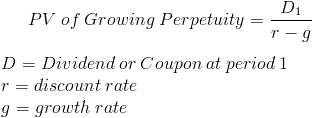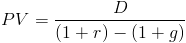# Present Value of Growing Perpetuity

PV of Growing Perpetuity Calculator (Click Here or Scroll Down)The present value of a growing perpetuity formula is the cash flow after the first period divided by the difference between the discount rate and the growth rate.

A growing perpetuity is a series of periodic payments that grow at a proportionate rate and are received for an infinite amount of time. An example of when the present value of a growing perpetuity formula may be used is commercial real estate. The rental cash flows could be considered indefinite and will grow over time.

It is important to note that the discount rate must be higher than the growth rate when using the present value of a growing perpetuity formula. This is due to the present value of a growing perpetuity formula being an infinite geometric series as explained in one of the following sections. In theory, if the growth rate is higher than the discount rate, the growing perpetuity would have an infinite value.

## Example of the Present Value of Growing Perpetuity Formula

An example of the present value of a growing perpetuity formula would be an annual cash flow of \$1000 that will continue indefinitely. This cash flow is expected to grow at 5% per year and the required return used for the discount rate is 10%. The equation for this example of the present value of a growing perpetuity formula would bewhich would return a present value of \$20,000.

## How the Present Value of Growing Perpetuity Formula is derived?

A growing perpetuity is a series of periodic payments that continue indefinitely and grow at a proportionate rate. Therefore, the formula for the present value of a growing perpetuity can be shown asThis series will continue for an infinite amount of periods. This formula could be rewritten asThis is considered to be an infinite geometric series with a common ratio of
(1+g)/(1+r). Putting this formula into the infinite geometric series formula would result inThis formula could be shortened by multiplying it by (1+r)/(1+r), which is to multiply it by one. This would result inwhich could be further reduced to the present value of a growing perpetuity formula shown at the top of the page.

New to Finance?